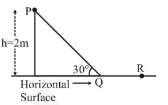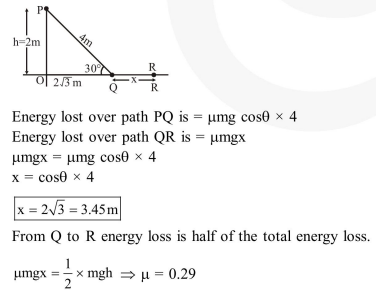Deepak Scored 45->99%ile with Bounce Back Crack Course. You can do it too!

# A point particle of mass,

Question:

A point particle of mass, moves along the uniformly rough track PQR as shown in the figure. The coefficient of friction, between the particle and the rough track equals $\mu$. The particle is released, from rest, from the point $\mathrm{P}$ and it comes to rest at a point $\mathrm{R}$. The energies, lost by the ball, over the parts, PQ and PR, of the track, are equal to each other, and no energy is lost when particle changes direction from $\mathrm{PQ}$ to $\mathrm{QR}$. The values of the coefficient of friction $\mu$ and the distance $x(=\mathrm{QR})$ are, respecitvely close to :1. $0.29$ and $6.5 \mathrm{~m}$

2. $0.2$ and $6.5 \mathrm{~m}$

3. $0.2$ and $3.5 \mathrm{~m}$

4. $0.29$ and $3.5 \mathrm{~m}$

Correct Option: , 4

Solution: